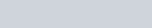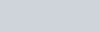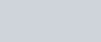Pump shaft radial thrust alternative calculations (in Imperial dimensions) 13-2

In past papers I have given you the formulas for calculating the radial thrust force on an end suction centrifugal pump shaft. It sometimes becomes confusing when you read other publications and find alternative formulas. Which ones are the best, and are they all correct?

The fact is they;re all close enough for the work we do in the field, so here are a couple of more to add to your collection. Use those formulas that you find the most convenient and less complicated.

If you want to approximate the shaft radial thrust at shut off conditions (the pump is running and the discharge valve is shut or almost shut), use the following formula:If you do not have shut off conditions, the following formula is a good approximationwhereRso= Radial thrust (in pounds) at shut off.

R = Radial thrust (in pounds) at operating conditions.

Kso= Thrust factor at shut off (see figure #1 below )

K = Thrust factor at operating conditions (see above formula).

Hso= Total head at shut off (in feet).

H = Total head at operating conditions (in feet).

sg.= Specific gravity of the liquid.

D2= Impeller diameter (in inches).

B2= Impeller width at the discharge including shrouds (in inches).

Q = Capacity at operating conditions (in gpm.).

Qn = Capacity at the best efficiency point (in gpm.).

x = An exponent varying between 0.7 and 3.3 established by testing. You probably do not have actual test data so you can safely assume a linear path between 0.7 at a specific speed of 500 and 3.3 at a specific speed of 3500. Check my Technical paper Volume 9 Number 12 if you are not familiar with the term specific speed.

PLEASE NOTE: Dand Dare not the same thing. D2 means to multiply D by its self (52 = 25). D2 means that particular D because sometimes (not in these examples) there is more than one D (shaft diameter) in the equation.

FIGURE #1### Posted

• On February 18, 2018